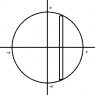# Trouble visualizing what is going on. Volume of object

• Jbreezy
So basically what they are saying is that the integral is the area of the cross section multiplied by the height of the section, and they are trying to solve for height. I guess what I am trying to do is integrate dy/dx from -r to r to find the height. I am not sure if that is what they are asking though.f

## Homework Statement

Hello,
Here is a link to a pdf .http://www.mrskeller.net/documents/hwsols14.pdf
I'm having issues with number 56. The solution makes no sense to me.

## The Attempt at a Solution

So, I did graph the equation y = sqrt(r^2 - x^2) and I understand that height of the circle. I understand that you need to integrate from -r to r. But I do not understand the integral by any means.

They have ##\int 4(r^2-x^2)) dx ## from -r to r
I thought that maybe there were doing something of the form ∏∫y^2 because that is what this chapter uses. But I guess not. They say that the length of a side is 2y = 2sqrt(r^2 - x^2). And the only way that I can see how to get from that equation to the integral they suggest is ly squaring 2y = 2sqrt(r^2 - x^2). So I don't understand that even because if you squared it and used the integral of the form ∏∫y^2 dx then your 4 dissapears. I just don't understand what is going on with this problem. Thanks

The volume of a solid with a known cross section is basically
$${\int_a}^b A(x) dx$$,
where A(x) represents the area of the cross section.

I believe the attached graphic illustrates the problem. Imagine a square lying on its side, the side being the thin rectangle in the diagram. Now imagine a bunch of thin vertical rectangles going from left to right (from -r to r). The distance from the x-axis to the top of this particular rectangle is y. Then you know the length of this thin rectangle (= the length of the square cross section), which is 2y. The area of the square cross section is
$$A = s^2$$
(s is the length of the side),
so plug in 2y for s. Now, what does y equal in terms of x and r? Plug that in for y, and the resulting expression serves as the integrand for this problem.

#### Attachments

•Square_Cross_Section.png
1.2 KB · Views: 467
•1 person
OMG! Thank you that makes a lot more sense. A lot more!